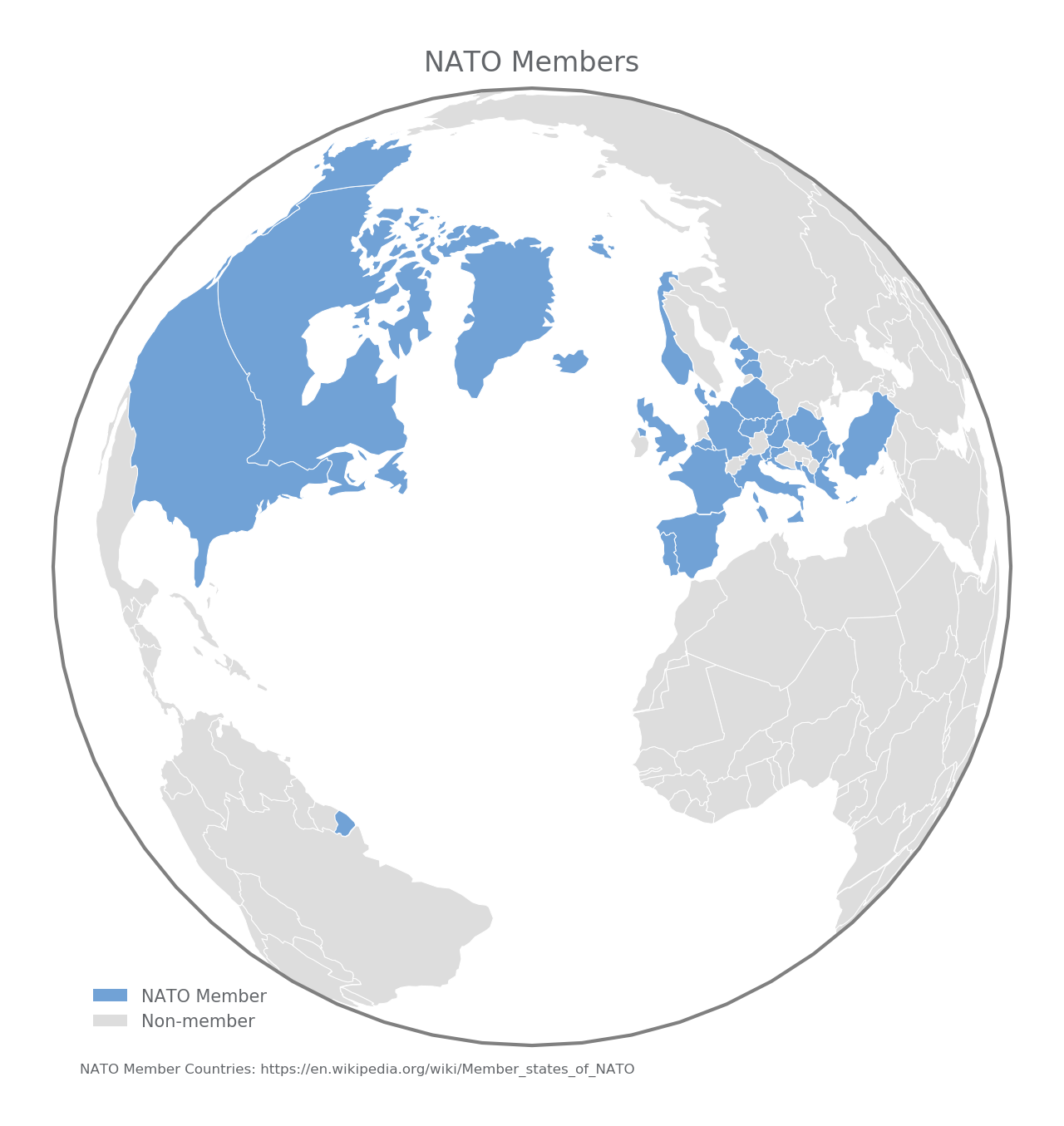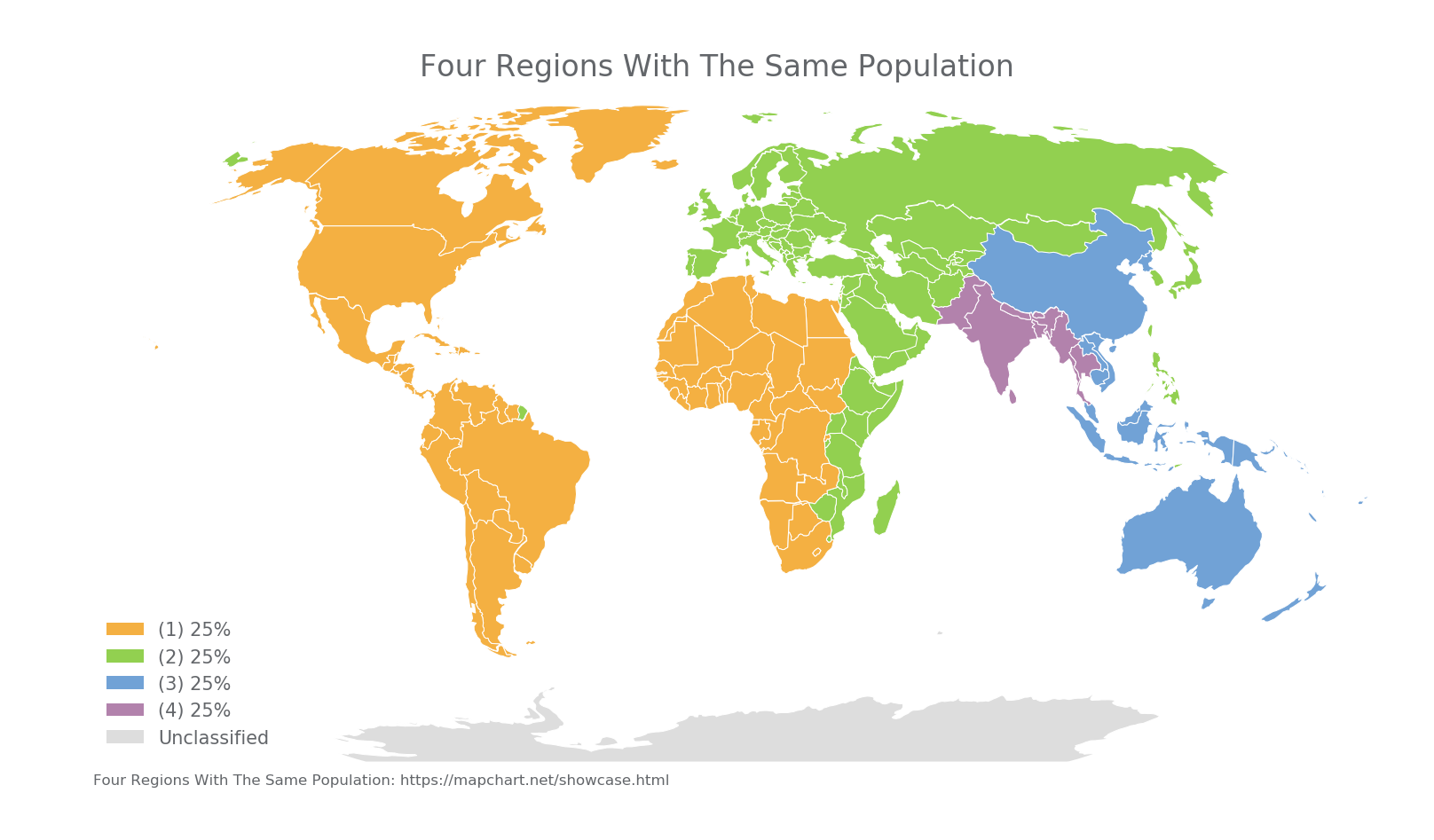# Highlighting Countries With CartopyHere is how to highlight select countries with Cartopy. (You can also highlight select states with Cartopy.)

Define which countries to highlight in countries.csv, and run cartopy_countries.py:

import matplotlib.pyplot as plt
import cartopy
import cartopy.crs as ccrs
import pandas as pd

def plot_countries(df,projection,colors,annotation,title,edgecolor):

ax = plt.axes(projection=projection)
ax.outline_patch.set_edgecolor(edgecolor)

category='cultural',
values = list(df[title].unique())

for country in countries:

# get classification
try:
classification = df.loc[country.attributes[attribute]][title]
except:
pass

facecolor=(colors[values.index(classification)]),
label=country.attributes[attribute],
edgecolor='#FFFFFF',
linewidth=.25)

# legend
import matplotlib.patches as mpatches
handles = []
for i in range(len(values)):
handles.append(mpatches.Rectangle((0, 0), 1, 1, facecolor=colors[i]))
plt.legend(handles, values,
loc='lower left', bbox_to_anchor=(0.025, -0.0),
fancybox=True, frameon=False, fontsize=5)

# annotate
ax.annotate(annotation, xy=(0, 0),  xycoords='figure fraction',
xytext=(0.0275, -0.025), textcoords='axes fraction',
horizontalalignment='left', verticalalignment='center', fontsize=4,
)

plt.title(title, fontsize=8)

title = 'maps/'+title+'.png'
print('Saved: {}'.format(title))

def main():

projection = ccrs.Robinson()
title = 'Four Regions With The Same Population'
colors = ['#f4b042', '#92D050','#71a2d6','#b282ac','#DDDDDD']
#colors = ['#orange' ,'#green','#blue ','#purple','#grey  ']
annotation = 'Four Regions With The Same Population: https://mapchart.net/showcase.html'
plot_countries(df,projection,colors,annotation,title,edgecolor='white')

projection = ccrs.Orthographic(-30,40)
colors = ['#71a2d6','#DDDDDD']
annotation = 'NATO Member Countries: https://en.wikipedia.org/wiki/Member_states_of_NATO'
title = 'NATO Members'
plot_countries(df,projection,colors,annotation,title,edgecolor='grey')

projection = ccrs.Orthographic(10,50)
colors = ['#000099','#DDDDDD']
annotation = 'EU Member Countries: https://en.wikipedia.org/wiki/Member_state_of_the_European_Union'
title = 'EU Members'
plot_countries(df,projection,colors,annotation,title,edgecolor='grey')

print('Done.\n')

if __name__ == '__main__':
main()


Two interesting examples are to show EU Members (above) or NATO members (below).Another interesting example is the world split into four regions with the same population (idea from mapchart.net).Note: I don't know how to highlight France without highlighting French Guiana (in South America). If you do, let me know.

© 2005 Matthew Kudija | Source